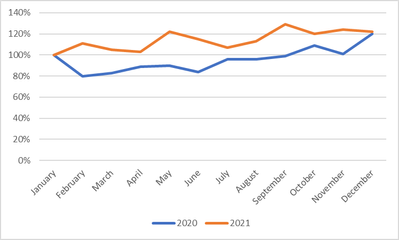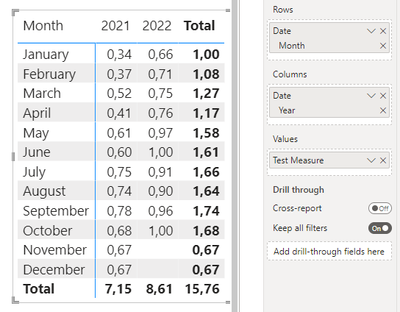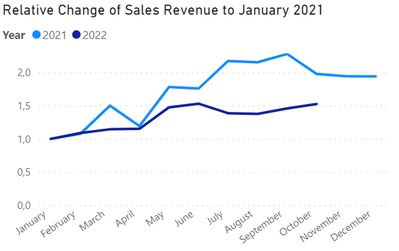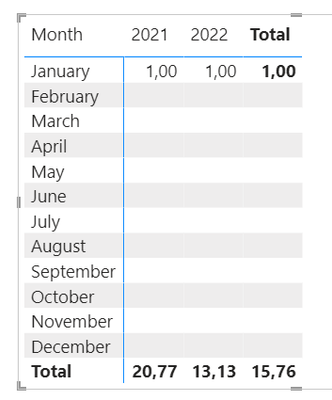cancel
Showing results for
Did you mean:

Fabric is Generally Available. Browse Fabric Presentations. Work towards your Fabric certification with the Cloud Skills Challenge.Helper III

## Have calculate distinguish column values

Hi everyone

In one of my dashboards, I am attempting to create a graph that looks as follows:The idea is to compare the performance over the course of a year of multiple years.

To do that, I have set up the following visual:My current measure looks as follows:

``````Test Measure =
var curr =
CALCULATE(
sum('Table'[SalesTotalEUR]),
KEEPFILTERS(
'Table'[Date]
)
)

var refer =
CALCULATE(
sum('Table'[SalesTotalEUR]),
FILTER(
ALL('Date'[Date]),
Month('Date'[Date])=1
)
)

var div=
DIVIDE(
curr,
refer,
""
)

return div ``````

My problem is that I am unable to keep my measure restricted to the year column. Therefore, all my January values (be they from 2021 or from 2022) are being used in my calculations. Can anyone therefore please tell me how to set up my measure so that it distinguished between the column values?

1 ACCEPTED SOLUTIONHelper III

So, after some more researching and plenty of trials and errors, I was finally able to find the correct dax command for the problem It is as follows:

``````Test Measure =

var yearselected=
SELECTEDVALUE('Date'[Date].[Year])

var curr =
sumx('Table', 'Table'[SalesTotalEUR])

var refer =
CALCULATE(
sum('Table'[SalesTotalEUR]),
FILTER(
ALL('Date'[Date]),
Month('Date'[Date])=1 && year('Date'[Date])=yearselected
)
)

var div=
DIVIDE(
curr,
refer,
""
)

return div``````

As you can see, as SELECTEDVALUES that has to be brought in as a variable is needed.

The resulting visual looks as desired:6 REPLIES 6Helper III

So, after some more researching and plenty of trials and errors, I was finally able to find the correct dax command for the problem It is as follows:

``````Test Measure =

var yearselected=
SELECTEDVALUE('Date'[Date].[Year])

var curr =
sumx('Table', 'Table'[SalesTotalEUR])

var refer =
CALCULATE(
sum('Table'[SalesTotalEUR]),
FILTER(
ALL('Date'[Date]),
Month('Date'[Date])=1 && year('Date'[Date])=yearselected
)
)

var div=
DIVIDE(
curr,
refer,
""
)

return div``````

As you can see, as SELECTEDVALUES that has to be brought in as a variable is needed.

The resulting visual looks as desired:Super User

You shouldn't need a new measure to generate a chart like this. Put Month on the x-axis and Year in the Legend box like this:Helper III

Hi @AlexisOlson ,

thanks for your reply. How would you set up your example to have your Sales Amount values normalized to 100 in January of every year? My goal is to show the relative change of my sales figures over the course of a year (e.g. sales grew by 10% in February 2021 (i.e. line goes to 110) while sales in February 2022 decreased by 6% (i.e. line goes to 94)). You can see what I mean in the graph above.

How would you approach this?Super User

Ah, I missed that detail. Try this:

``````Normalized Sales =
DIVIDE (
[Sales Amount],
CALCULATE (
[Sales Amount],
REMOVEFILTERS ( 'Date'[Month Number] ),
'Date'[Month] = "January"
)
)``````

The removes the month name and month number filter context without removing the year filter context.Helper III

Hi @AlexisOlson ,

unfortunately, this only fills out the January cells in my tableI have slightly modified your command in order to fit my tables

``````Test 3 =
DIVIDE (
sum('Table'[SalesTotalEUR]),
CALCULATE (
sum('Table'[SalesTotalEUR]),
REMOVEFILTERS ( 'Date'[Month Number] ),
'Date'[Date].[Month] = "January"
),
""
)``````

Any idea how to have the CALCULATE section of your solution expanded to all cells?Super User

I'm guessing your model doesn't have exactly the same columns with the same names as the file I was testing with. Do you have columns for month name, month number, and year in your date table? If so, what are they? If not, then my solution clearly won't work since it's referencing non-existing things.Announcements#### Power BI Monthly Update - November 2023

Check out the November 2023 Power BI update to learn about new features.#### Fabric Community News unified experience

Read the latest Fabric Community announcements, including updates on Power BI, Synapse, Data Factory and Data Activator.#### The largest Power BI and Fabric virtual conference

130+ sessions, 130+ speakers, Product managers, MVPs, and experts. All about Power BI and Fabric. Attend online or watch the recordings.Top Solution Authors
Top Kudoed Authors
Users online (4,677)